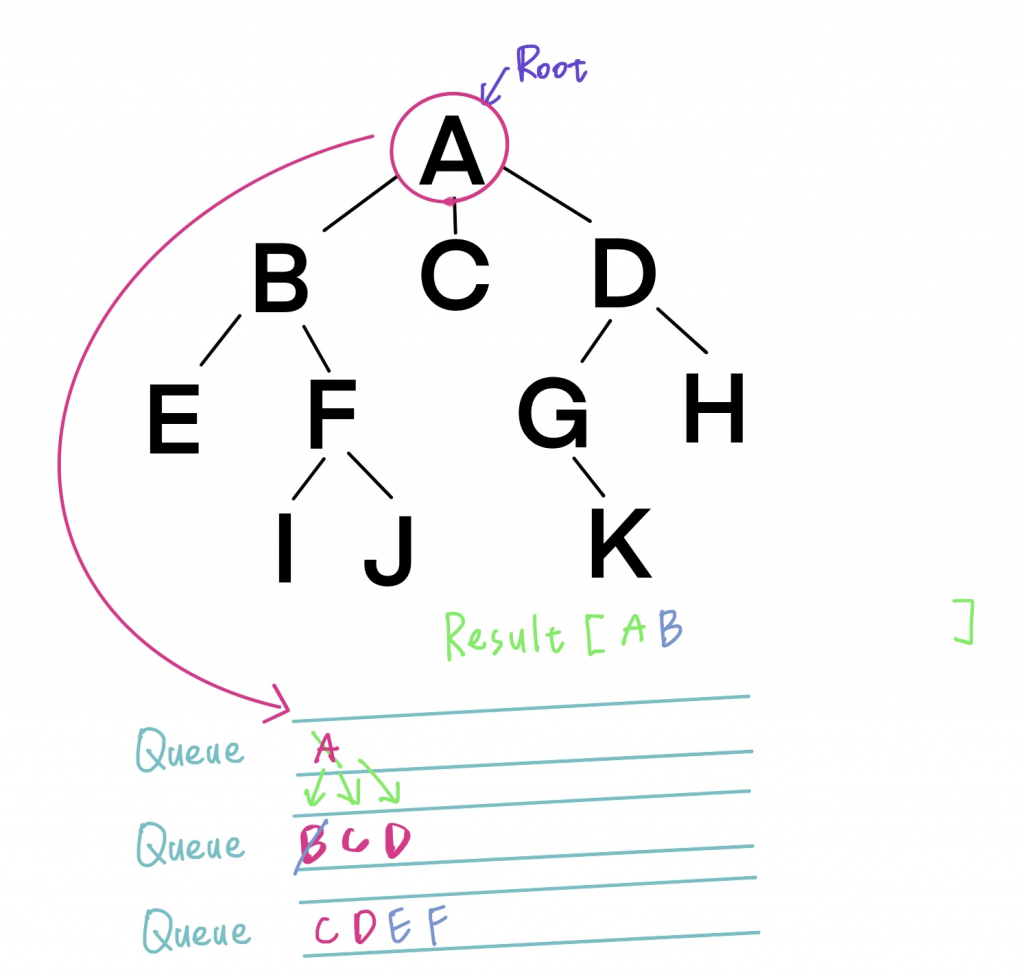# Day 21 ：广度优先搜寻 Breadth-First search(BFS)

Queue(伫列)是先进来的元素先出去(First In First Out = FIFO)的资料结构，通常用於让程序具有排队功能，依序执行工作，``````/**
* Definition for a binary tree node.
* function TreeNode(val, left, right) {
*     this.val = (val===undefined ? 0 : val)
*     this.left = (left===undefined ? null : left)
*     this.right = (right===undefined ? null : right)
* }
*/
/**
* @param {TreeNode} root
* @return {number[][]}
*/

var levelOrder = function(root) {
if (!root) return [];

const res = [];
const q = [];
q.push(root);

while(q.length) {
const lvl = [];
const size = q.length;

for (let i = 0; i < size; i++) {
const node = q.shift();
lvl.push(node.val);
if (node.left) q.push(node.left);
if (node.right) q.push(node.right);
}
res.push(lvl);
}
return res;
};
``````

##### Android Studio初学笔记-Day14-Switch和Toggle Button

Switch和Toggle Button 今天要讲两个简单的元件，在还没碰到这两个元件之前，想要做到...